Computational & Technology Resources an online resource for computational,engineering & technology publications not logged in - login Civil-Comp ProceedingsISSN 1759-3433 CCP: 83PROCEEDINGS OF THE EIGHTH INTERNATIONAL CONFERENCE ON COMPUTATIONAL STRUCTURES TECHNOLOGY Edited by: B.H.V. Topping, G. Montero and R. Montenegro Paper 183Simulation of the Load-Bearing Capacity of Structures Using Fuzzy Random Processes B. Möller, W. Graf, A. Hoffmann and J.-U. SickertDepartment of Civil Engineering, Institute of Statics and Dynamics of Structures, Technical University Dresden, Germany doi:10.4203/ccp.83.183 Full Bibliographic Reference for this paper B. Möller, W. Graf, A. Hoffmann, J.-U. Sickert, "Simulation of the Load-Bearing Capacity of Structures Using Fuzzy Random Processes", in B.H.V. Topping, G. Montero, R. Montenegro, (Editors), "Proceedings of the Eighth International Conference on Computational Structures Technology", Civil-Comp Press, Stirlingshire, UK, Paper 183, 2006. doi:10.4203/ccp.83.183 Keywords: fuzzy stochastic finite element method, fuzzy random process, uncertainty, load-bearing capacity, RC-structures. Summary Structures are subjected to time-variant stresses such as static and dynamic loads or environmental conditions. Furthermore, as a result of load-dependent damage and load-independent alteration, resistance of structures is also time-dependent. Stress and resistance depend on parameters which are more or less characterized by data uncertainty. From this follows, that the time-dependent structural reactions and the time-dependent load-bearing capacity of structures are also uncertain. The appropriate numerical prognosis of the uncertain time-dependent load-bearing capacity is only possible if the load-bearing behavior is simulated with the aid of physically and geometrically nonlinear algorithms considering the data uncertainty. In order to consider the time-dependencies and the data uncertainty within the numerical simulation the new mathematical model fuzzy random processes is introduced. Fuzzy random processes are special fuzzy random functions with time-dependency. Using fuzzy random functions data uncertainty can be described which is characterized by fuzzy randomness . Fuzzy randomness arises if the stochastic description of uncertain parameters is additionally informally or lexically uncertain. Fuzzy random functions describe fuzzy random fluctuations, which depend on external conditions. External conditions include, for example, time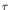, spatial coordinates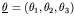, air pressure or temperature, which are lumped together in the parameter vector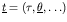. A fuzzy random function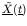is defined as family of fuzzy random variables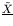for fixed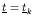in the extended probability space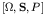explained in . In order to take account of fuzzy random functions the fuzzy stochastic structural analysis is developed . With the fuzzy stochastic structural analysis uncertain time-dependent input processes are mapped onto uncertain time-dependent resulting processes. Because of modeling the input processes as fuzzy random processes the resulting processes are also fuzzy random processes. The fuzzy stochastic structural analysis comprises the parts fuzzy analysis, stochastic analysis, and deterministic fundamental solution. The deterministic fundamental solution complies with the deterministic numerical simulation of the nonlinear structural behavior. In engineering the finite element method (FEM) is the most powerful tool to simulate the nonlinear structural behavior. In order to combine the fuzzy stochastic structural analysis and the FEM efficiently the fuzzy stochastic finite element method (FSFEM) has been developed. In the FSFEM, random processes and fuzzy processes are considered as special cases of fuzzy random processes. The comprehensive analysis concept of the FSFEM is formulated as further development of introduced probabilistic approaches which are known as stochastic finite element method. The numerical solution of the FSFEM is based on the discretization of the fuzzy random input processes in a set of fuzzy correlated fuzzy random variables . Every fuzzy random variable is characterized by their fuzzy probability distribution function. The fuzzy correlation is quantified by fuzzy correlation functions. Both the fuzzy probability distribution functions and the fuzzy correlation functions are represented by means of fuzzy bunch parameters. From this it follows that the fuzzy probability distribution functions and the fuzzy correlation functions can be numerically outlined as a fuzzy set of real-valued probability distribution functions as well as real-valued correlation functions. All fuzzy bunch parameters combined with the Cartesian product constitute the space of fuzzy bunch parameters. The space of bunch parameters is mapped onto the fuzzy bunch parameters of the fuzzy random resulting processes. The mapping procedure is based on the fuzzy analysis algorithm which replaces the extension principle by an optimization problem. Inside of the fuzzy analysis a stochastic analysis as the Monte-Carlo simulation (MCS) and the deterministic fundamental solution are processed. The samples computed with the MCS are evaluated statistically. The algorithms are demonstrated by the way of an example using the new multi-reference plane model to compute the nonlinear behavior of RC structures . References 1 B. Möller, M. Beer, "Fuzzy-Randomness - Uncertainty in Civil Engineering and Computational Mechanics", Berlin: Springer-Verl., 2004. 2 J.-U. Sickert, M. Beer, W. Graf, B. Möller, "Fuzzy Probabilistic Structural Analysis considering Fuzzy Random Functions" In: Der Kiureghian, A.; Madanat, S.; Pestana, J.M. (Eds.), "Proc. 9th Int. Conf. On Applications of Statistics and Probability in Civil Engineering", San Francisco, Rotterdam: Millpress, 2003, pp. 379-386. 3 B. Möller, W. Graf, A. Hoffmann, F. Steinigen, "Numerical simulation of RC structures with textile reinforcement". Computers & Structures, Vol. 83 (2005) 19-20, pp. 1659-1688. doi:10.1016/j.compstruc.2004.11.024 purchase the full-text of this paper (price £20) Back to top ©Civil-Comp Limited 2020 - terms & conditions